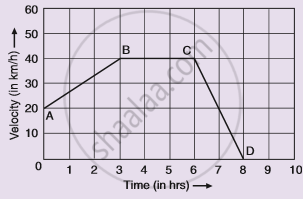# Given Alongside is the Velocity-time Graph for a Moving Body : - Science

Graph

Given alongside is the velocity-time graph for a moving body :
Find :
(i) Velocity of the body at point C.
(ii) Acceleration acting on the body between A and B.
(iii) Acceleration acting on the body between B and C.#### Solution

(i) BC represents uniform velocity. So velocity of the body at point C is 40 km
(ii) Acceleration = Slope of line AB
= ((40-20))/((3-0)) (km)/(hr^2)
= 6.66  "km/hr"^2
(iii) BC represents uniform velocity, so, acceleration acting on the body is zero.

Concept: Velocity - Time Graphs
Is there an error in this question or solution?

#### APPEARS IN

Lakhmir Singh Science Class 9 Physics
Chapter 1 Motion
Hots Questions | Q 56 | Page 43